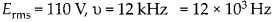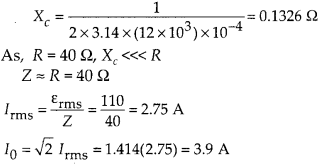Enlightened

# Question 16: NCERT Solutions for 12th Class Physics: Chapter 7-Alternating Current

• 0

Question 16: NCERT Solutions for 12th Class Physics: Chapter 7-Alternating Current

Obtain the answer to (a) and (b) in Q.15 if the circuit is connected to a 110 V, 12 kHz supply? Hence, explain the statement that a capacitor is a conductor at very high frequencies. Compare this behaviour with that of a capacitor in a dc circuit after the steady state.

Share

1. Solution:
Given,(a)This value of current is same as that without capacitor in the circuit. So, at high frequency, a capacitor offer negligible resistance (0.1326 Ω in this case), it behave like a conductor.

(b)In dc circuit, after steady state, v = 0 and accordingly, XC = ∞, i.e., a capacitor amounts to an open circuit, i.e., it is a perfect insulator of current.

Check the complete chapter with solutions.

NCERT Solutions for 12th Class Physics: Chapter 7-Alternating Current

• 0